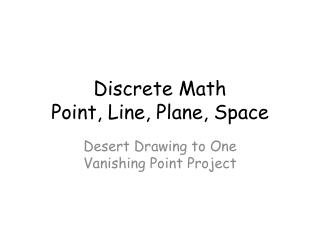DownloadDownload PresentationDiscrete Math Point, Line, Plane, Space

# Discrete Math Point, Line, Plane, Space

Télécharger la présentation## Discrete Math Point, Line, Plane, Space

- - - - - - - - - - - - - - - - - - - - - - - - - - - E N D - - - - - - - - - - - - - - - - - - - - - - - - - - -
##### Presentation Transcript

1. Discrete MathPoint, Line, Plane, Space Desert Drawing to One Vanishing Point Project

2. Point • In Discrete Geometry, a point is a dot.

3. Point • The ancient Greeks idealized points as an exact location, having no size or shape.

4. Point • In Coordinate Geometry: points are ordered pairs.

5. Point • A fourth description of point is of a node or a vertex in a network.

6. Point • Points can make continuous lines.

7. Point • Between every point there is always another point.

8. Point • Between every point, there is an infinite numberof points.

9. Point • A point cannot be defined or drawn, but only visualized with a model.

10. Line • A line is determined by two points.

11. Line • In a plane, a line can: intersect another line, be parallel to another line, or be coincident to this line.

12. Line • In space, a line can: intersect another line, be parallel to another line, be coincident to another line, or be skew to another line.

13. Line • A line cannot be defined or drawn, but only visualized with a model.

14. Plane • A plane is determined by three non-collinear points.

15. Plane • When two planes intersect, they form a line.

16. Plane • A plane cannot be defined or drawn, but only visualized with a model.

17. Space • Space is the set of all points.

18. Space • When all points in space are collinear, the geometry is one-dimensional.

19. Space • When all points in space are coplanar, the geometry is two-dimensional (2D) or plane geometry.

20. Space • Other figures, such as spheres, boxes, cones, and other tangible objects do not lie in one plane and are three-dimensional or 3D. The study of these is called solid geometry.

21. Space • Space cannot be defined or drawn, but only visualized with a model.

22. Discrete Geometry • Models of points: • Dot matrix printers • Displays made with LEDs • Circular metal pipes arranged in hexagonal prisms • Some paintings • Wildflowers in bloom

23. Models of Points

24. Models of Points

25. Models of Points

26. Models of Points

27. Models of Points

28. Euclid’s 5 Postulates • To draw a straight line from any point to any point.

29. Euclid’s 5 Postulates 2. To produce a finite straight line continuously in a straight line.

30. Euclid’s 5 Postulates 3. To describe a circle with any center and distance.

31. Euclid’s 5 Postulates 4. That all right angles are equal to one another.

32. Euclid’s 5 Postulates 5. That, if a straight line falling on two straight lines make the interior angles on the same side less than two right angles, the two straight lines, if produced indefinitely, meet on that side on which are the angles less than the two right angles.

33. Euclid to Ptolemy • When Ptolemy asked if there was an easier way to learn geometry Euclid replied: "There is no royal road to Geometry."

34. Perspective • Drawing in Perspective • Although mathematicians don't often draw in perspective, the concept and terminology are important.

35. Perspective • A perspective drawing gives a two-dimensional object a feeling of depth.

36. Perspective • Often one thinks of the artist's or observer's eye as this vanishing point and sketches lines of sight to connect them.

37. Perspective • Objects can be drawn in one- two- or three-point perspective, depending on how many vanishing points are used.

38. Perspective • Parallel horizontal and vertical lines go to their own vanishing point, depending on their relationship to each other.

39. Perspective • Multiple vanishing points should line up on the vanishing line which corresponds with the horizon line at the height of the observer's eye.

40. Perspective • Parallel lines now meet in the distance at a vanishing point.

41. Perspective • Mathematicians typically draw non-perspective drawings, utilizing dashed or dotted hidden lines to indicate parts not normally seen.

42. Perspective

43. Non - Perspective

44. Non - Perspective

45. A Contraction Drawing

46. Non - Perspective

47. Anamorphosis

48. Perspective

49. Perspective • Desert Drawing

50. Perspective • Desert Drawing